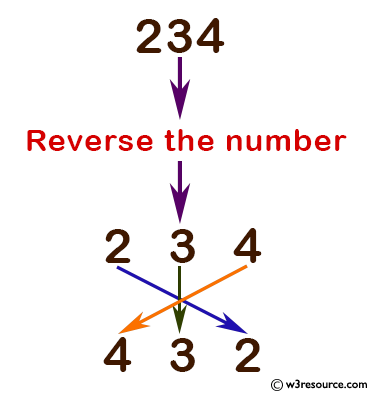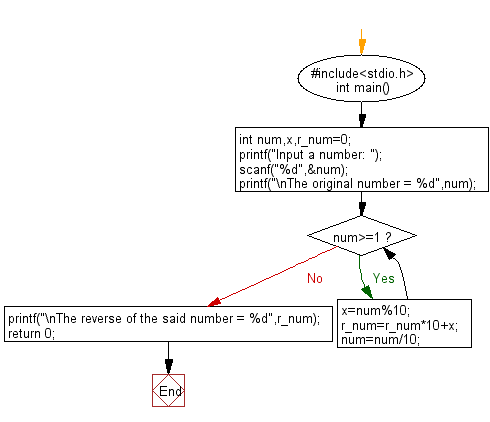﻿ C Program: Reverse and print a given number - w3resource

# C Exercises: Reverse and print a given number

## C Basic Declarations and Expressions: Exercise-57 with Solution

Write a C program to reverse and print a given number.

Pictorial Presentation:Sample Solution:

C Code:

``````#include<stdio.h>
int main() {
int num, x, r_num = 0;

// Prompt user to input a number
printf("Input a number: ");
scanf("%d", &num);

// Display the original number
printf("\nThe original number = %d", num);

// Reverse the digits of the number
while (num >= 1) {
x = num % 10;
r_num = r_num * 10 + x;
num = num / 10;
}

// Display the reverse of the number
printf("\nThe reverse of the said number = %d", r_num);

return 0;
}
``````

Sample Output:

```Input a number:
The original number = 234
The reverse of the said number = 432
```

Flowchart:C programming Code Editor:

What is the difficulty level of this exercise?

Test your Programming skills with w3resource's quiz.

﻿